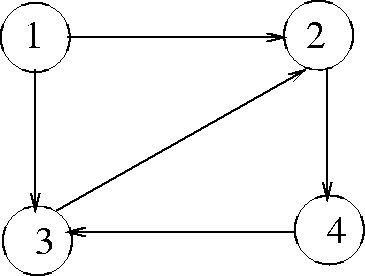Next:7.1.1 Data Structures for Graph RepresentationUp:7. Directed GraphsPrevious:7. Directed Graphs

# 7.1 Directed Graphs

A directed graph or digraph G comprises

1. a set of vertices V

2. a set of directed edges or arcs, E (an arc is an ordered pair of vertices)

Example: See Figure 7.1V = {1, 2, 3, 4}

E = {(1,2), (1,3), (2,4), (3,2), (4,3)}

• If there is an arc (v, w), we say w is adjacent to v.
• A path is a sequence of vertices v1, v2,...vn such that the vertex pairs (v1, v2),(v2, v3), ..., (vn - 1, vn) are arcs in the graph. The length of a path is the number of arcs on the path. A single vertex v by itself denotes a path of length zero.
• A path v1, v2,..., vn is said to be simple if the vertices are distinct, except possibly v1 and vn. If v1 = vn and n > 1, we call such a path a simple cycle.Next:7.1.1 Data Structures for Graph RepresentationUp:7. Directed GraphsPrevious:7. Directed Graphs
eEL,CSA_Dept,IISc,Bangalore